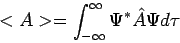# Operator Terminology: What Does the Asterisk Mean?

• Nezva
In summary, the asterisk means that the complex conjugation of a function is being used. When substituting the psi function into the expectation value, the asterisk is used to denote the complex conjugation. When expanding the eigenfunctions in the position basis, the asterisk is used to denote the complex conjugation of the eigenfunction.

#### NezvaWhat does the asterisk mean?

The asterisk stand for complex conjugation.

In the case of $$\Psi=c$$1$$\Psi$$1$$+ c$$2$$\Psi$$2$$+ ... + c$$n$$\Psi$$n

And the operator A(hat) => A(hat)$$\Psi$$1 = a1$$\Psi$$1; A(hat)$$\Psi$$2 = a2$$\Psi$$2; A(hat)$$\Psi$$n = an$$\Psi$$n

Calculate: $$\left\langle\Psi\left|A(hat)\right|\right\Psi\rangle$$

Nezva said:
Calculate: $$\left\langle\Psi\left|A(hat)\right|\right\Psi\rangle$$

Are you giving me a pop quiz?You need to show us an attempt in order to receive help with your homework problem. Simply stating the problem does not qualify as an attempt.

I'm trying to substitute the psi function into the expectation value. I do not understand how to use the asterisk in this case. Especially if the constants in the function are complex... I'm trying to understand the concept and am unsure what to ask.

$$\int$$ c*n$$\Psi$$n$$\left| A(hat) \right| c$$n$$\Psi$$n

Nezva said:
I'm trying to substitute the psi function into the expectation value. I do not understand how to use the asterisk in this case. Especially if the constants in the function are complex... I'm trying to understand the concept and am unsure what to ask.

Okay, well when you say:

Nezva said:
In the case of $$\Psi=c$$1$$\Psi$$1$$+ c$$2$$\Psi$$2$$+ ... + c$$n$$\Psi$$n

Do you mean $|\Psi\rangle=c_1|\psi_1\rangle+c_2|\psi_2\rangle+\ldots+c_n|\psi_n\rangle$ (abstract form), or do you mean $\Psi(\textbf{r})=c_1\psi_1(\textbf{r})+c_2\psi_2(\textbf{r})+\ldots+c_n\psi_n(\textbf{r})$ (all the wavefunctions are expanded in the position basis)?

More importantly, what do you know about $\{|\psi_1\rangle,|\psi_2\rangle,\ldots,|\psi_n\rangle\}$? For example, are they orthogonal? Normalized?

The $$\Psi$$is linear combination of n orthonormal eigenfunctions of the linear operator, A(hat).

What effect does a normalized function vs a 'orthonormal' function have on the 'expectation value'? Sorry I'm being thrown into this terminology very rudely. Any help is greatly appreciated.

Orthonormal means that the eigenfunctions are both orthogonal and normalized to unity.

In abstract form this means that $\langle\psi_i|\psi_j\rangle=\delta_{ij}$, where $$\delta_{ij}=\left\{\begin{array}{lr} 0, & i\neq j \\ 1, & i=j \end{array}\right.[/itex] is the Kronecker delta. When you expand the eigenfunctions in the position basis (i.e. $\psi(\textbf{r})=\langle\hat{\mathbf{r}}|\psi\rangle$), you get [tex]\langle\psi_i|\psi_j\rangle=\int_{-\infty}^{\infty}\psi_i^{*}(\textbf{r})\psi_j(\textbf{r})d\tau=\delta_{ij}$$

Start by calculating $\hat{A}|\Psi\rangle$...what do you get for that?